# Everyday Mathematics Lattice Multiplication Lattice Multiplication Lattice multiplication

• Slides: 14Everyday Mathematics Lattice Multiplication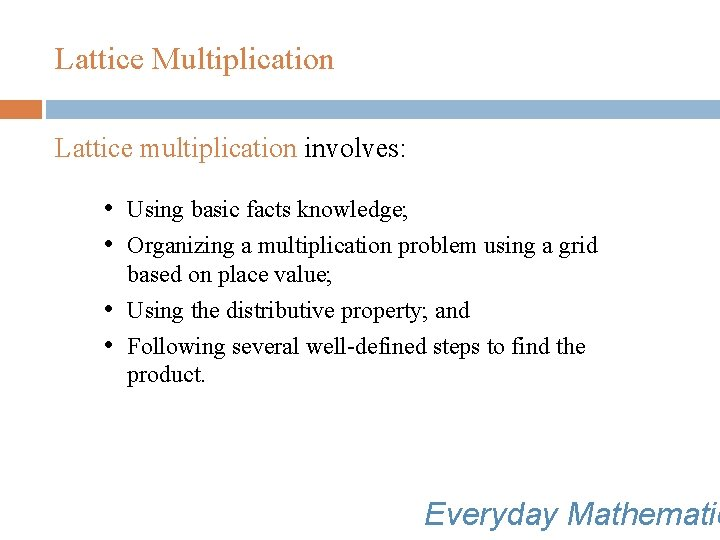Lattice Multiplication Lattice multiplication involves: • Using basic facts knowledge; • Organizing a multiplication problem using a grid based on place value; • Using the distributive property; and • Following several well-defined steps to find the product. Everyday Mathematic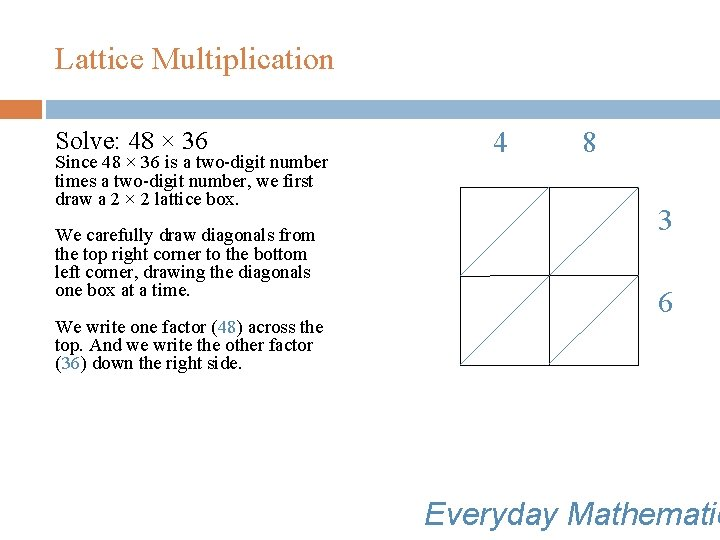Lattice Multiplication Solve: 48 × 36 Since 48 × 36 is a two-digit number times a two-digit number, we first draw a 2 × 2 lattice box. We carefully draw diagonals from the top right corner to the bottom left corner, drawing the diagonals one box at a time. We write one factor (48) across the top. And we write the other factor (36) down the right side. 4 8 3 6 Everyday Mathematic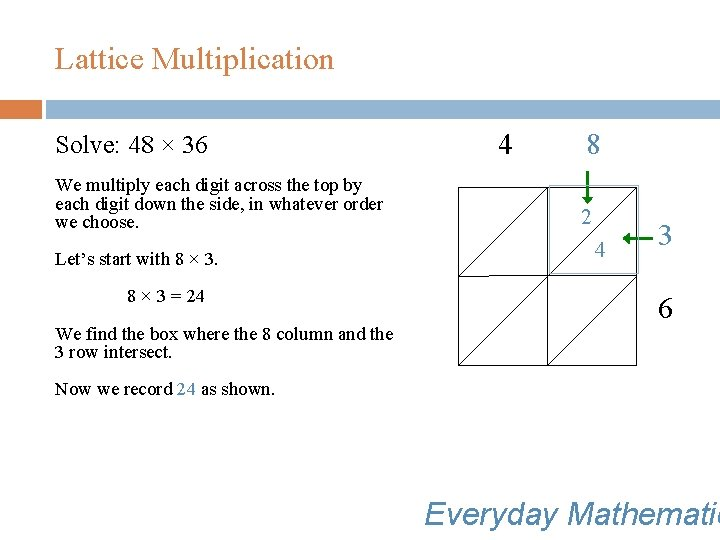Lattice Multiplication Solve: 48 × 36 We multiply each digit across the top by each digit down the side, in whatever order we choose. Let’s start with 8 × 3 = 24 We find the box where the 8 column and the 3 row intersect. 4 8 2 4 3 6 Now we record 24 as shown. Everyday MathematicLattice Multiplication Solve: 48 × 36 4 × 3 = 12 We find the box where the 4 column and the 3 row intersect. We record the 12 as shown. 4 1 8 2 2 4 3 6 Everyday MathematicLattice Multiplication Solve: 48 × 36 8 × 6 = 48 We find the box where the 8 column and the 6 row intersect. We record the 48 as shown. 4 8 2 1 2 4 4 8 3 6 Everyday Mathematic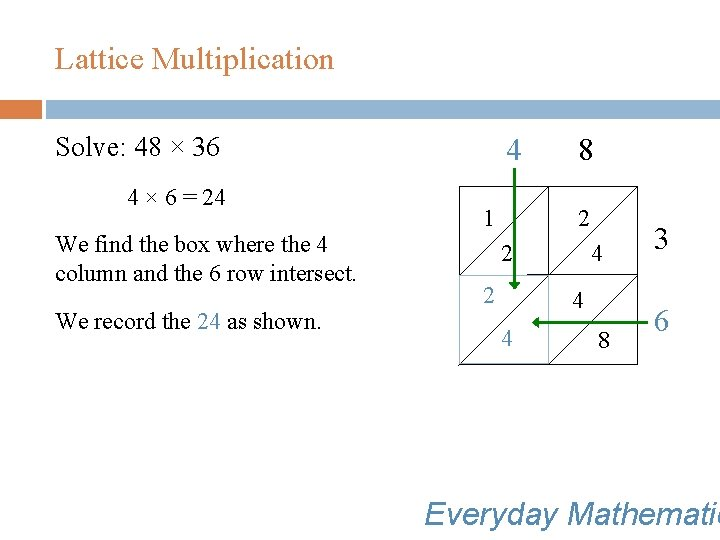Lattice Multiplication Solve: 48 × 36 4 × 6 = 24 We find the box where the 4 column and the 6 row intersect. We record the 24 as shown. 4 1 8 2 2 2 4 4 4 8 3 6 Everyday MathematicLattice Multiplication Solve: 48 × 36 4 The diagonals separate the digits by place-value columns. The next step is to add along the diagonals. We start with the ones. There are 8 ones. We record 8 ones. 8 1 s s u ho 2 2 d an t 4 2 4 4 ds e dr 8 3 6 n hu 8 s ten s e on Everyday Mathematic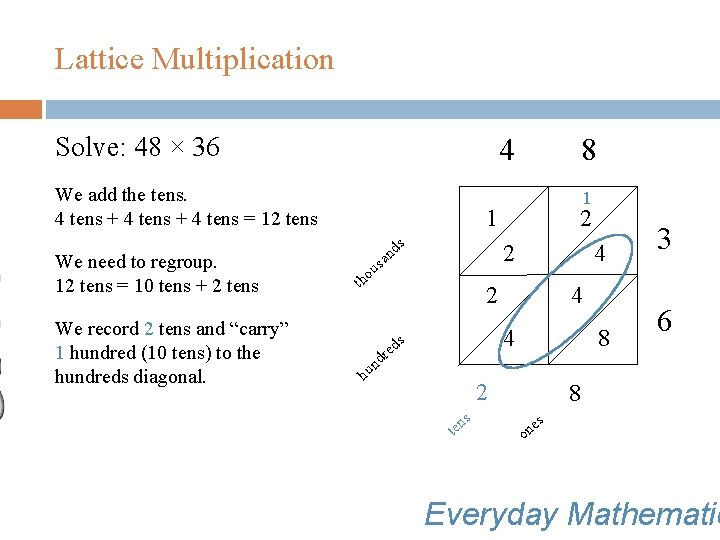Lattice Multiplication Solve: 48 × 36 4 We add the tens. 4 tens + 4 tens = 12 tens We need to regroup. 12 tens = 10 tens + 2 tens We record 2 tens and “carry” 1 hundred (10 tens) to the hundreds diagonal. 8 1 1 s s u ho 2 2 d an t 4 2 4 4 ds e dr 8 3 6 n hu 2 s ten 8 s e on Everyday MathematicLattice Multiplication Solve: 48 × 36 4 We add the hundreds. 2 hundreds + 1 hundred 7 hundreds We record 7 hundreds. 8 1 1 s s u ho 2 2 d an t 4 2 7 4 4 ds e dr 8 3 6 n hu 2 s ten 8 s e on Everyday MathematicLattice Multiplication Solve: 48 × 36 4 We add the thousands. 1 1 s There is 1 thousand. s u ho 2 2 d an t 4 2 7 We record 1 thousand. 8 4 4 ds e dr 8 3 6 n hu 2 s ten 8 s e on Everyday Mathematic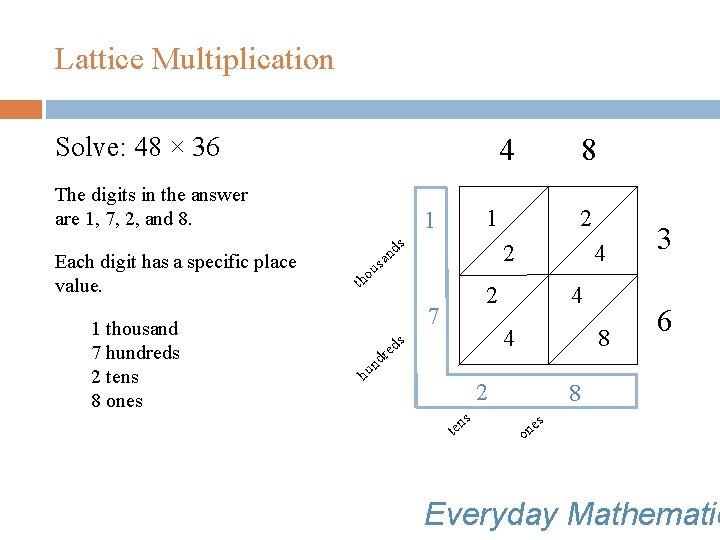Lattice Multiplication Solve: 48 × 36 4 The digits in the answer are 1, 7, 2, and 8. Each digit has a specific place value. 1 thousand 7 hundreds 2 tens 8 ones 1 1 s s u ho 8 2 2 d an t 4 2 7 4 4 ds e dr 8 3 6 n hu 2 s ten 8 s e on Everyday MathematicLattice Multiplication Solve: 48 × 36 The final answer is 1, 728, because 1 thousand + 7 hundreds + 2 tens + 8 ones = 1, 728. The product of 48 × 36 is 1, 728. Everyday Mathematic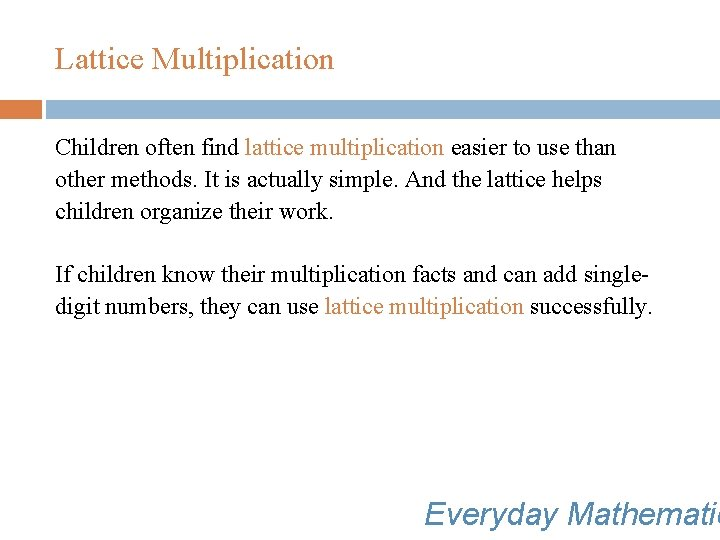Lattice Multiplication Children often find lattice multiplication easier to use than other methods. It is actually simple. And the lattice helps children organize their work. If children know their multiplication facts and can add singledigit numbers, they can use lattice multiplication successfully. Everyday Mathematic